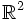# Difference between revisions of "Tour:Examples peek two (beginners)"

PREVIOUS: Mind's eye test two (beginners) |UP: Introduction two (beginners) | NEXT: Interdisciplinary problems two (beginners)

The page develops some examples of groups, monoids and other related structures in different contexts. The page can be skipped without any loss and content in this page will not be referred to except in future Examples peek pages. This page develops examples originally looked at in Examples peek one.

## Integers under multiplication

1. Prove that the rational numbers, under multiplication, form a monoid. Further, prove that the nonzero rational numbers form a subgroup of this monoid.
2. Prove that the positive integers, under multiplication, form a cancellative monoid.
3. For any positive integer$n$, prove that the set of positive integers$a$ such that$n | a - 1$ is a submonoid of the monoid of all positive integers.
4. Using the identity$(a^2 + b^2)(c^2 + d^2) = (ad + bc)^2 + (ac - bd)^2$, prove that the set of all integers that can be written as a sum of two squares, forms a monoid under multiplication.

1. Prove that the nonnegative integers form a monoid under addition.
2. Prove that for any positive integer$n$, the set of integers$m \ge n$, along with 0, form a submonoid of this monoid.
3. NEEDS LOT OF THOUGHT: Let$a,b$ be positive integers whose greatest common divisor is 1. Prove that the set of elements of the form$ax + by$, where$x,y$ are nonnegative integers, is a submonoid of the monoid of nonnegative integers. Further, prove that this submonoid is cofinite: there are only finitely many nonnegative integers not in this submonoid.

## Modular arithmetic

In examples peek one, we defined the additive group of integers mod$n$, for any positive integer$n$. Now, we define the multiplicative monoid of integers mod$n$, for any positive integer$n$.

1. For a positive integer$n$, consider the set$\{ 0,1,2,3,\dots,n-1\}$. Define a multiplication on the set by the following rule: multiple them as integers, and then take the remainder of the product mod$n$. Prove that with this multiplication, we get a monoid.
2. Prove that this monoid is never a group.
3. Find all the idempotent elements in this monoid. In other words, find all the elements$a$ satisfying$a^2 = a$. The solution depends, of course, on$n$.
4. Prove that the number of$x$ such that$x^2 = 1$ in this monoid is 1 for$n = 1$ and$n = 2$, 2 for$n = 4$,$n = 2p^k$ and$n = p^k$ where$k$ is an odd prime, and strictly greater than 2 otherwise.
5. Prove that the cancellative elements of this monoid form a subgroup, and these are the same as the elements$a$ that are relatively prime to$n$.

## Permutations and functions

1. Let$S$ be an infinite set. Prove that the set of all functions from$S$ to$S$ form a monoid under composition. Here, the product of two functions$f$ and$g$ is the function$f \circ g = x \mapsto f(g(x))$. We'll call this monoid$M$.
2. Prove that the set of injective maps from$S$ to$S$ is a submonoid, the set of surjective maps from$S$ to$S$ is a submonoid, and the set of bijective maps from$S$ to$S$ is a subgroup (it is the group of all permutations on$S$, denoted$\operatorname{Sym}(S)$.
3. Prove that if$f:S \to S$ is injective, then$f$ is a left-cancellative element of the monoid of all functions under composition.
4. NEEDS SOME THOUGHT: Prove the converse statement: if$f:S \to S$ is left-cancellative, then$f$ is injective.
5. (For those who know the Cantor-Bernstein-Schroeder theorem) Prove that the left-invertible elements in$M$ are precisely the injective maps.
6. Formulate and prove analogous statements related surjective maps, right-cancellative elements and right-invertible elements.

## Metric spaces and maps between them

1. Let$M$ be the set of all functions$f:\R^2 \to \R^2$ with the property that for any$x,y \in \R^2$, the distance between$f(x)$ and$f(y)$ is not greater than the distance between$x$ and$y$. Prove that$M$ is a monoid under function composition.
2. NEEDS SOME THOUGHT: Find an element of$M$ that gives a surjective map that is not injective.
3. Prove that the group of invertible elements of$M$ is precisely the group of isometries of$\R^2$.
This page is part of the Groupprops Guided tour for beginners (Jump to beginning of tour). If you found anything difficult or unclear, make a note of it; it is likely to be resolved by the end of the tour.
PREVIOUS: Mind's eye test two (beginners) | UP: Introduction two (beginners) | NEXT: Interdisciplinary problems two (beginners)